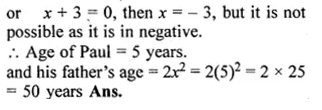Guru

# (i) The sum of the ages of Vivek and his younger brother Amit is 47 years. The product of their ages in years is 550. Find their ages. (ii) Paul is x years old and his father’s age is twice the square of Paul’s age. Ten years hence, the father’s age will be four times Paul’s age. Find their present ages.

• 0

This question has been taken from Book:- ML aggarwal, Avichal publication, class10th, quadratic equation in one variable, chapter 5, exercise 5.5
This is an important ques and asked in 2017

(i) The sum of the ages of Vivek and his younger brother Amit is 47 years. The product of their ages in years is 550. Find their ages.

(ii) Paul is x years old and his father’s age is twice the square of Paul’s age.

Ten years hence, the father’s age will be four times Paul’s age.

Find their present ages.
Question no. 42, ML Aggarwal, chapter 5, exercise 5.5, quadratic equation in one variable, ICSE board,

Share

1. Solution:
(i) Let Vivek’s present age be x years.
His brother’s age = (47 – x) years
According to question,
x(47 – x) = 550
⇒ 47x – x² = 550
⇒ x² – 47x + 550 = 0
⇒ x² – 25x – 22x + 550 = 0
⇒ x(x – 25) – 22(x – 25) – 0• 0